# Summary

It will be shown, that Mitscherlich's formula, the crop yield as function of the fertilizer, can be partitioned into two parts. One comes from the fertilizer in the soil, the other from the fertilizer, supplied from outside. As a consequence, the quantity of the fertilizer, which is extracted from the soil by the crop, can be computed. Correspondingly new fertilizing can be planned.

# Introduction

One problem in fertilizing, on which many authors have worked, recently was reported on by Giebel et al. (2006): "Until now one may be able to derive a prognosis upon the spatial average of soil mineral-N content of a field. However, no approaches are available that would allow the estimation of the spatial distribution of plant-available N over an entire field within the same or a following year. Nevertheless, adequate representation of spatial N distribution within a field is important to site-specific and environmentally sustainable N fertilization". This result of spatial averages of soil mineral-N content of a field was obtained by Giebel et al. with samples of ground-drillings and a following analysis.

Another problem is the question, what part of the crop-yield comes from the plant-available fertilizer in the soil, and what part is caused by the given fertilizer. This problem is solved here in quite a different way without technical devices, only with analytical methods and the fact, that the Mitscherlich- process can be partitioned into two exponential processes. One of these processes is the so-called standardized Mitscherlich process, the other is an exponential dying process. From the quantity of lost fertilizer in the soil consequences for future fertilizing can be drawn.

The Law of Mitscherlich (1909) is an exponential growing process with horizontal asymptote. This sort of processes is widely known in natural sciences: In biology we find it as Mendel's law of genetics, in mechanics it is the generalization of Hooke's law (Schneeberger 2005): the first (linear) term of the Taylor-series of this generalization is Hooke's law; in electrical engineering charging of a battery follows this law, etc.

Mitscherlich first formulated the law in form of a differential equation: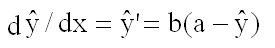(1)

where x is the quantity of fertilizer, y the crop-yield and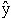(as usual in statistics) the hypothetical value of y; a is the maximum value of the yield (we exclude over-fertilization!) and b>0 is the most important exponential factor. Formula (1) means, that the growing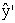of the cropis proportional to (a-). With boundary condition(x = 0) = c we have as solution of equation (1)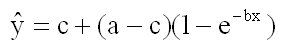(2)

The boundary condition(x = 0) = c means, that c is the crop-yield with only the plant-available fertilizer in the soil, with no supplementary fertilizer, i.e. x = 0. See the curvein figure 1.

# Materials and Methods

For our analysis we use the results of an experiment with winter-wheat at the University of Technology München/Weihenstephan in 2002, given in table 1: For every of the r=15 values xr of fertilizer, s=4 values of crop-yield yrs are given. For clarity in the figures not the experimental points (xr, yrs) with the great variance of the y-values, but (xr,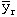) are given as stars; see also the meansin table 1.

 r 1 2 3 4 5 6 7 8 9 10 11 12 13 14 15 s xr 0 80 100 120 140 160 160 180 180 200 200 220 220 240 260 yrs 1 40.8 73.8 79.6 84.5 97.7 82.9 96.2 102.6 102.8 96.4 103.5 98.6 102.7 102.2 108.1 2 58.6 89.9 94.7 78.1 105.5 89.4 92.7 102.1 92.1 102.1 94.8 103.5 105.0 104.7 106.5 3 62.7 76.4 84.4 78.2 80.4 109.0 97.6 103.4 104.7 102.0 104.8 93.7 104.0 97.7 110.0 4 51.8 79.3 88.5 94.1 93.4 96.4 93.5 92.5 96.1 108.4 103.1 107.4 104.6 78.0 103.353.475 79.850 86.800 83.725 94.250 94.425 95.000 100.150 98.925 102.225 101.550 100.800 104.075 95.650 106.975

The three parameters a,b,c of the Mitscherlich-curve (2) are estimated with the method of Least Squares of Gauss, i.e. a, b and c are solutions of the condition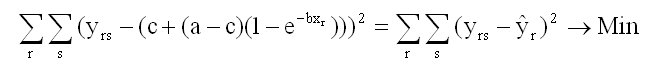(3)

This problem is solved iteratively with the non-linear Simplex-Method of Nelder and Mead (1965). The result is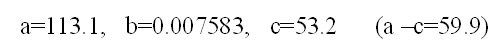(4)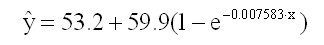(5)

It can easily be shown, that computation with the values of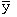(instead of y) yields the same result.

The curve, plotted in figure 1, is extrapolated to negative values of x (dotted line). We get x = d = -83.8 as solution of(x) = 0. -d(>0) is the plant-available fertilizer in the soil, which gives the corresponding crop-yield=c=53.2, i.e. without fertilizing from outside. With this soil-immanent fertilizer -d we can write formula (2) also in the form of the Mitscherlich-Baule function (Baule 1918). See also Frank, Beattie and Embleton (1990):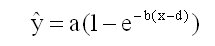(6)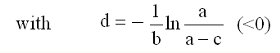(7)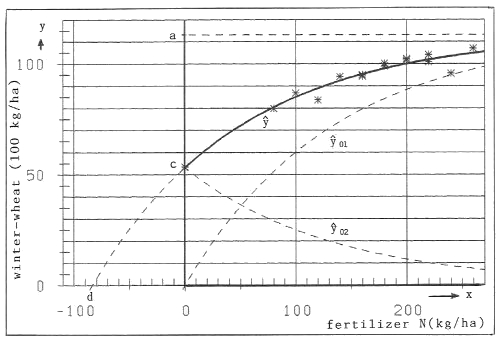Figure 1. Partition of the Mitscherlich-curveinto the two parts01 and02

The following theorem immediately results from formula (6):

Theorem: The general Mitscherlich-curve(x) of formula (6) with -d>0, or formula (2) with c>0, originates from the "standardized" Mitscherlich curve01(x)=a(1-e-bx) with d=0 in formula (6) or c=0 in formula (2) by simply shifting the curve01(x) to the left for -d >0 units (of variable x). See figure 1.

For the strategy of fertilizing in the future, to avoid too much or too less fertilizing with the known consequences, it is important to state, that according to Liebig's Law we have for the total quantity q of plant-available fertilizer in the soil: q≥-d

## Partition of Mitscherlich's Formula

We can write formula (2) in the form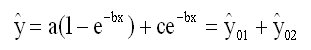(8)

The parts01 and02 are of specific importance:

a)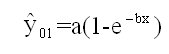(9)

is the standardized Mitscherlich process, beginning at the origin (x,)=(0,0), i.e. c=0. So this process is generated alone by the fertilizer, supplied from outside.

b)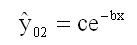(10)

is a diminishing exponential process, starting from02(x=0)=c. So02(x) is the crop-yield, caused by the plant-available fertilizer in the soil alone. Note the remark to formula (2)!

Remark: Processes of the form (b) are the most important "dying processes" in natural sciences (e.g. radioactive decay), biology , medicine, economics, etc. Here one better would speak of an exhaustion process (of the fertilizer in the soil).

In figure 1 the curves01 and02 are plotted as dashed lines.

# Results and discussion

If the quantity of fertilizer from outside is zero (i.e. x=0), we have01=0 and the crop is=02=c=53,2 (100 kg/ha of winter-wheat), which comes alone from the fertilizer in the soil -d=83.8 (kg/ha of N).

Now we assume, that for example a quantity of fertilizer x = x0=100 kg/ha of N is given from outside. Then we get from formula (9) the quantity of crop01(x0), which comes from the given x0 and with formula (10) we get the quantity of crop02(x0), which comes from the fertilizer in the soil. The total crop is(x0)=01(x0)+02(x0) We get01(x0)=60,1 |02(x0)=24,9 |(x0)=85,0

The problem now is: What quantity of fertilizer in the soil gives the crop02(x0)? This is found with the auxiliary Mitscherlich process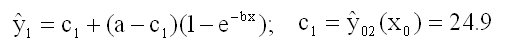(11)

which yields x=d1=-32,8 as solution of1(x)=0. -d1=32.8 (kg/ha) is the soil-immanent plant-available part of fertilizer, which, together with the fertilizer x0=100 (kg/ha) from outside, yields the total crop(x0)=85,0 (100 kg/ha). See figure 2.
Note: Equation (11) can also be written in the equivalent form: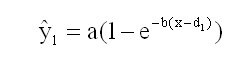(11a)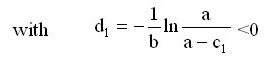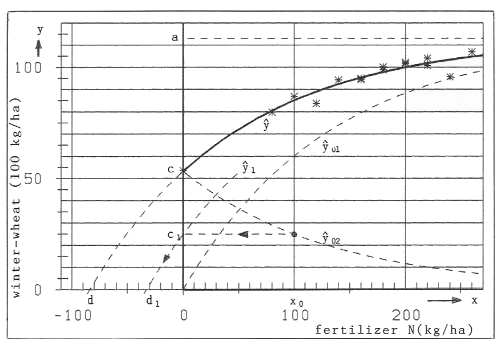Figure 2: Example of fertilizing with x0=100 kg/ha of N. Calculation of the quantity of fertilizer -d1=32,8 kg/ha of N, supplied by the soil

A necessary supplement follows with Paper 2 (Paper 2: Mitcherlich's Law: A Supplement)

# Acknowledgement

I have to thank Dr. Maidl of the University of Technology München (Weihenstephan)/Germany for providing me with the data, used in this article.

# References

Baule, B. (1918). Zu Mitscherlichs Gesetz der physiologischen Beziehungen, Landwirtschaftliche Jahrbücher 51, 363-385.

Frank M.D.,Beattie B.R. and Embleton M.E. (1990). A Comparison of Alternative Crop Response Models, American Journal of Agricultural Economics, 72, 597-603.

Giebel, A. et al.(2006). How representatively can we sample soil mineral nitrogen? Journal of Plant Nutrition and Soil Sciences, 169, 52-59.

Mitscherlich, E.A.(1909). Das Gesetz des Minimums und das Gesetz des abnehmenden Bodenertrags, Landwirtschaftliche Jahrbücher, 38, 537-552.

Nelder, J.R. and Mead, R. (1965). A Simplex Method for function minimization. The Computer Journal, 7, 303-313.

Schneeberger, H. (2005). Theory of the stress-strain relationship of concrete and steel. In R.K.Dhir, M.D.Newlands and L.J.Csetenyi (eds.): Applications of Nanotechnology in Concrete Design. Proceedings of the International Conference at the University of Dundee, Scotland , UK, 105-112.

Download this Paper in PDF format: Mitscherlich's Law: Sum of two exponential Processes - Conclusions (PDF, 0,5 MB)